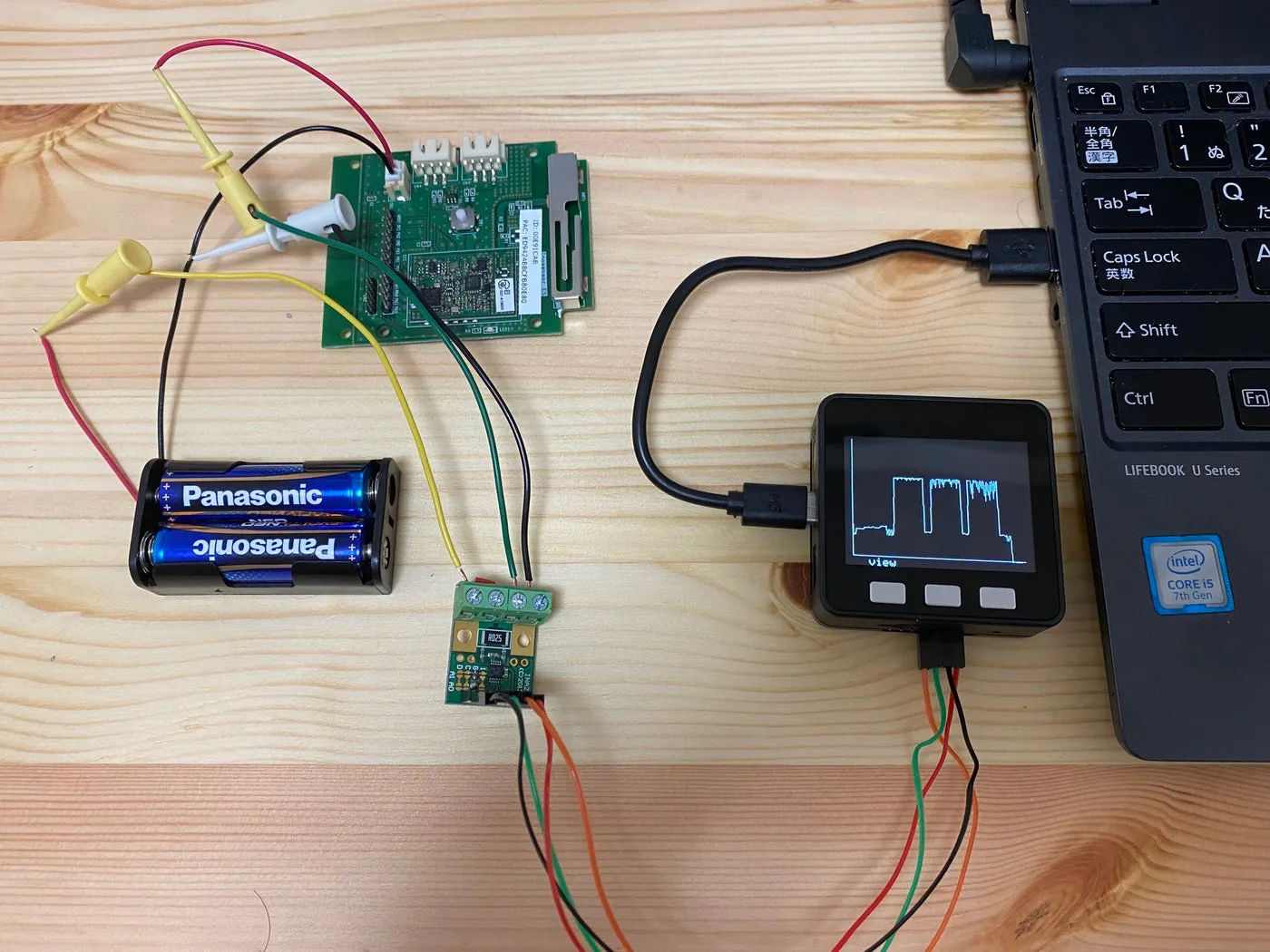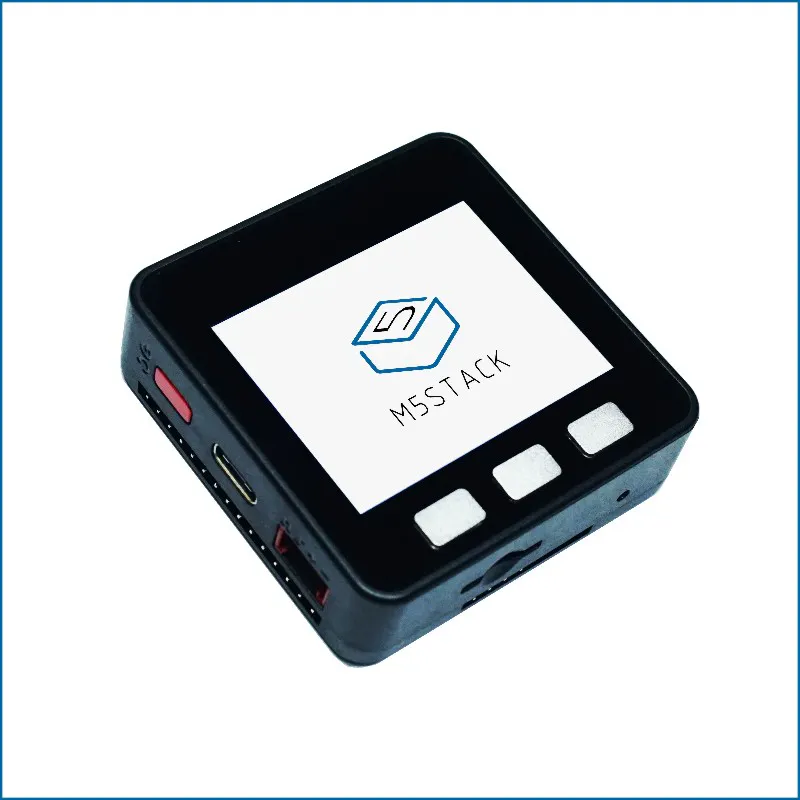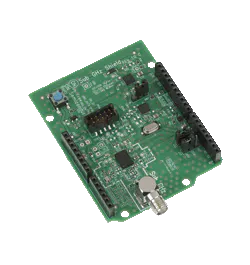# Use M5Stack to Measure Power Consumption

Thanks to @ghibi for providing material. This project will measure the power consumption of the Sigfox module (IFS-M01) with M5Stack.

IntermediateFull instructions provided2 hours1,744## Things used in this project

### Hardware componentsM5Stack ESP32 Basic Core IoT Development Kit
×1
 INA226 PRC voltmeter
×1onsemi EU-SIGFOX-GEVB
×1

## Code

### Untitled file

C/C++
```#include <M5Stack.h>
#include <Wire.h>
#include "INA226PRC.h"

INA226PRC ina226prc;

void beep(int freq, int duration, uint8_t volume);

#define TIMER0 0
hw_timer_t * samplingTimer = NULL;

#define NSAMPLES 3000     // 4ms x 3000 = 12秒

short ampbuf[NSAMPLES];
short voltbuf[NSAMPLES];

int sampling  = 4;  // Sampling interval (ms)
int startthreshold = 3;  // Current value to start recording (mA)

volatile int t0flag;

void IRAM_ATTR onTimer0() {
t0flag = 1;
}

int selectitem(int *candi, int items, int val, char *tail) {
int focused;

for (int i = 0; i < items; i++) {
if (candi[i] == val) {
focused = i;
}
}
M5.Lcd.fillScreen(BLACK);
bool first = true;
while (true) {
bool modified = false;
M5.update();
if (M5.BtnA.wasPressed()) {
focused--;
modified = true;
}
if (M5.BtnC.wasPressed()) {
focused++;
modified = true;
}
if (M5.BtnB.wasPressed()) {
M5.Lcd.fillScreen(BLACK);
return candi[focused];
}
if (first || modified) {
first = false;
beep(1000, 100, 2);
for (int i = 0; i < items; i++) {
M5.Lcd.setCursor(100, 40 + i * 20);
int16_t textcolor = ((focused % items) == i) ? BLACK : WHITE;
int16_t backcolor = ((focused % items) == i) ? WHITE : BLACK;
M5.Lcd.setTextColor(textcolor, backcolor);
M5.Lcd.printf(" %d ", candi[i]);
M5.Lcd.setTextColor(WHITE, BLACK);
M5.Lcd.print(tail);
}
}
}
}

void config() {
int focused = 2;
const int nItems = 3;
int thresholds[] = {2, 3, 5, 10, 20};
int samplings[] = {2, 4, 10, 20, 50};
bool first = true;
while (true) {
bool modified = false;
M5.update();
if (M5.BtnA.wasPressed()) {
focused--;  // up button
modified = true;
}
if (M5.BtnC.wasPressed()) {
focused++;  // down button
modified = true;
}
if (M5.BtnB.wasPressed()) {  // change button
modified = true;
switch (focused % nItems) {
case 0:
startthreshold = selectitem(thresholds, sizeof(thresholds) / sizeof(int), startthreshold, "mA");
break;
case 1:
sampling = selectitem(samplings, sizeof(samplings) / sizeof(int), sampling, "ms");
break;
case 2:
default:
return;
}
}
if (first || modified) {  // Noise seems to come out from the speaker when I write in the loop
first = false;
beep(1000, 100, 2);
M5.Lcd.setCursor(20, 40);
M5.Lcd.print("Start threshold: ");
if ((focused % nItems) == 0) M5.Lcd.setTextColor(BLACK, WHITE);
M5.Lcd.printf(" %d ", startthreshold);
if ((focused % nItems) == 0) M5.Lcd.setTextColor(WHITE, BLACK);
M5.Lcd.print("mA");

M5.Lcd.setCursor(20, 100);
M5.Lcd.print("Sampling period: ");
if ((focused % nItems) == 1) M5.Lcd.setTextColor(BLACK, WHITE);
M5.Lcd.printf(" %d ", sampling);
if ((focused % nItems) == 1) M5.Lcd.setTextColor(WHITE, BLACK);
M5.Lcd.print("ms");

M5.Lcd.setCursor(20, 160);
if ((focused % nItems) == 2) M5.Lcd.setTextColor(BLACK, WHITE);
M5.Lcd.print(" DONE ");
if ((focused % nItems) == 2) M5.Lcd.setTextColor(WHITE, BLACK);
}
}
}

#define X0 10
#define Y0 220

void drawData(short maxamp) {
M5.Lcd.fillRect(0, 0, 320, 220, BLACK);
maxamp = ((maxamp / 100) + 1) * 100;
for (int i = 0; i < 299; i++) {
int y0 = map(ampbuf[i * 10], 0, maxamp, Y0, 0);
int y1 = map(ampbuf[(i + 1) * 10], 0, maxamp, Y0, 0);
M5.Lcd.drawLine(i + X0, y0, i + 1 + X0, y1, WHITE);
Serial.printf("%d, %d,  %d, %d\r\n", ampbuf[i * 10], ampbuf[(i + 1) * 10], y0, y1);
}
M5.Lcd.drawLine(X0, Y0, 310, Y0, WHITE);
M5.Lcd.drawLine(X0, 0, X0, Y0, WHITE);
}

void setup() {
Serial.begin(115200);
M5.begin();
Wire.begin();
ina226prc.begin();

Serial.print("Manufacture ID: ");

M5.Lcd.setTextSize(2);
config();

M5.Lcd.fillScreen(BLACK);
beep(1000, 100, 2);
M5.Lcd.setCursor(20, 100);
M5.Lcd.print("Press A button");
M5.Lcd.setCursor(40, 120);
M5.Lcd.print("to start sampling");

while (true) {
M5.update();
if (M5.BtnA.wasPressed()) break;
}

M5.Lcd.fillScreen(BLACK);
beep(2000, 100, 2);

samplingTimer = timerBegin(TIMER0, 80, true);  //Initialize 1 microsecond timer
timerAttachInterrupt(samplingTimer, &onTimer0, true);  // Set the interrupt handling function
timerAlarmWrite(samplingTimer, sampling * 1000, true);  // set timer value for sampling milliseconds

timerAlarmEnable(samplingTimer);  // Fire timer

bool started = false;
int indx = 0;
short maxamp = 0;

M5.Lcd.fillRect(50, 100, 200, 10, BLACK);
while (true) {
t0flag = 0;
while (t0flag == 0) {  // Wait for timer interrupt
delay(0);
}

if (!started) {
// If the current value is below the threshold (startthreshold), do not start measurement
if (amp * 0.1 > -(float)startthreshold && amp * 0.1 < (float)startthreshold) {
continue;
}
started = true;  // Start measurement when the current value exceeds the threshold
}
ampbuf[indx] = amp;  // Record current value in memory
voltbuf[indx] = volt;  // Record voltage value in memory
maxamp = max(amp, maxamp);
M5.Lcd.setCursor(100, 100);
M5.Lcd.print(indx * 100 / NSAMPLES); M5.Lcd.print(" %");
if (++indx >= NSAMPLES) {  // If the number of data exceeds the number of samples, end the cyclic processing
break;
}
}
timerAlarmDisable(samplingTimer);  // Stop the timer

M5.Lcd.fillScreen(BLACK);
M5.Lcd.setTextSize(2);
M5.Lcd.setCursor(20, 100);
beep(2000, 400, 2);

Serial.println("time, current(mA), volt(mV)");
for (int i = 0; i < NSAMPLES; i++) {
Serial.printf("%d, %.2f, %.2f\r\n", sampling * i, ampbuf[i] * 0.1, voltbuf[i] * 1.25);
}

M5.Lcd.setCursor(40, 160);
M5.Lcd.print("Press A button");
M5.Lcd.setCursor(40, 180);
M5.Lcd.print("to view data");

while (true) {
M5.update();
if (M5.BtnA.wasPressed()) {
drawData(maxamp);
}
}
}

void loop()
{
}
```

ghibi